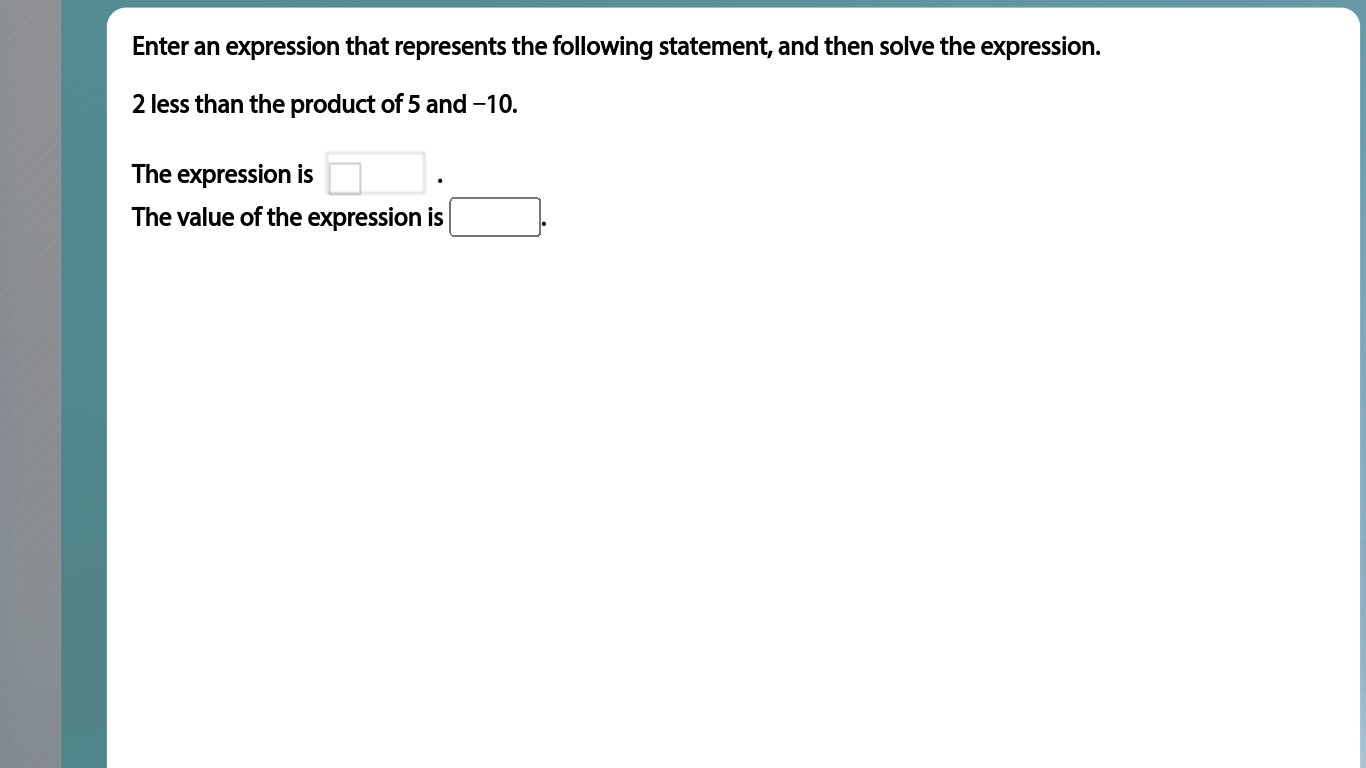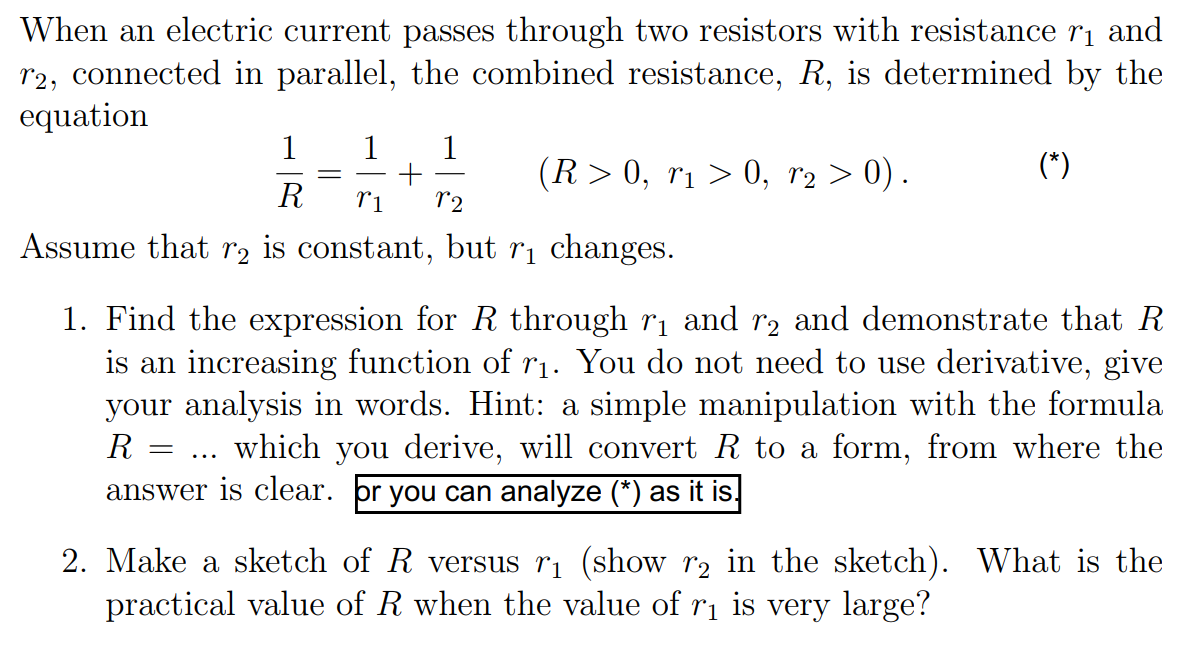# College math questions and answers

Recent questions in Post Secondaryseiyakou2005n1 2022-05-23 Answered

### In my differential equations book, I have found the following:Let ${P}_{0}\left(\frac{dy}{dx}{\right)}^{n}+{P}_{1}\left(\frac{dy}{dx}{\right)}^{n-1}+{P}_{2}\left(\frac{dy}{dx}{\right)}^{n-2}+......+{P}_{n-1}\left(\frac{dy}{dx}\right)+{P}_{n}=0$ be the differential equation of first degree 1 and order n (where ${P}_{i}$ $\mathrm{\forall }$ i $\in 0,1,2,...n$ are functions of x and y).Assuming that it is solvable for p, it can be represented as:$\left[p-{f}_{1}\left(x,y\right)\right]\left[p-{f}_{2}\left(x,y\right)\right]\left[p-{f}_{3}\left(x,y\right)\right]........\left[p-{f}_{n}\left(x,y\right)\right]=0$equating each factor to Zero, we get n differential equations of first order and first degree.Let the solution to these n factors be:Where ${c}_{1},{c}_{2},{c}_{3}.....{c}_{n}$ are arbitrary constants of integration. Since all the c’s can have any one of an infinite number of values, the above solutions will remain general if we replace ${c}_{1},{c}_{2},{c}_{3}.....{c}_{n}$ by a single arbitrary constant c. Then the n solutions (4) can be re-written asThey can be combined to form the general solution as follows:Now, my question is, whether equation (1) is the most general form of solution to the differential equation.I think the following is the most general form of solution to the differential equation :If (1) is the general solution, the constant of integration can be found out by only one IVP say, $y\left(0\right)=0$. So, one IVP will give the particular solution. If (2) is the general solution, one IVP might not be able to give the particular solution to the problem.Waylon Ruiz 2022-05-23 Answered

### Is there any simple proof (one that does not use continuous functional calculus) for the statement that $\sigma \left({x}^{\ast }x\right)\subseteq \left[0,\mathrm{\infty }\right)$ for any $x\in \mathcal{A}$ where $\mathcal{A}$ is a commutative ${C}^{\ast }$-Algebra?Aiden Barry 2022-05-23 Answered

### My book demonstrates how to test whether a set of vectors $S=\left\{{v}_{1},{v}_{2},{v}_{3}\right\}$ is linearly independent by writing ${c}_{1}{v}_{1}+{c}_{2}{v}_{2}+{c}_{3}{v}_{3}=0$, equating corresponding components to form a system of linear equations, and then reducing the augmented matrix of this system using Gauss-Jordan elimination.My question is, couldn't you just evaluate the determinant of the matrix rather than using elimination? Couldn't you say that if the determinant is nonzero, the system has only the trivial solution and therefore S is independent; and if it's zero, the system has infinitely many solutions (since we know it can't have no solutions as it has at least the trivial solution) and S is therefore dependent? Or do you have to use Gaussian elimination?kunyatia33 2022-05-23 Answered

### Given is the sequence ${x}_{1}=0,\phantom{\rule{thickmathspace}{0ex}}{x}_{n+1}=\sqrt{2+{x}_{n}}$. Prove:$\underset{n\to \mathrm{\infty }}{lim}{2}^{n}\sqrt{2-{x}_{n}}=\pi$Hint:Use the following formulas:$\mathrm{cos}\left(\frac{x}{2}\right)=\sqrt{\frac{1+\mathrm{cos}x}{2}}$$\mathrm{sin}\left(\frac{x}{2}\right)=\sqrt{\frac{1-\mathrm{cos}x}{2}}$Any idea how to solve this problem?Angel Malone 2022-05-23 Answered

### What is the polar form of $\left(216,-6\right)$ ?Isabela Sherman 2022-05-23 Answered

### How do you find the median of 37, 54, 50, 29, 34, 41?res2bfitjq 2022-05-23 Answered

### For $f\left(t\right)=\left(\frac{1}{t},-\frac{1}{{t}^{2}}\right)$ what is the distance between $f\left(2\right)$ and $f\left(5\right)$?cyfwelestoi 2022-05-23 Answered

### Combinatorial proof of ${P}_{r}^{n+1}=r!+r\left({P}_{r-1}^{n}+{P}_{r-1}^{n-1}+\cdots +{P}_{r-1}^{r}\right)$, where ${P}_{r}^{n}$ denotes the number of r- permutations of an n element set.groupweird40 2022-05-23 Answered

### At school, or in a first-year course on DEs, we learn (perhaps in less abstract language) that if you have a linear $n$th-order differential equation$Ly=f$then the general solution is something of the form$y={a}_{1}{y}_{1}+...+{a}_{n}{y}_{n}+g$where the ${y}_{i}$ are independent and satisfy $L{y}_{i}=0$, and $g$ satisfies $Lg=f$. Then we receive lots of training in how to find the ${y}_{i}$ and $g$.Obviously any choice of the ${a}_{i}$ will give us a solution to $Ly=f$. But how do you know that there aren't any more solutions?We justify this by making an analogy with systems of linear equations $Ax=b$, saying something along the lines of 'the space of solutions has the same dimension as the kernel of $A$'. But that works in finite dimensions - how do we know that the same is true with linear operators?shelohz0 2022-05-23 Answered

### Let $ß=\left({b}_{1},...,{b}_{n}\right)$ be a basis of the vector space $V$, let $T:V\to V$ be a linear transformation of $V$, and let B be the $ß$-matrix of $T$.(a) Prove that $v\in \mathrm{ker}\left(T\right)$ if and only if $\left[v{\right]}_{B}\in \mathrm{ker}\left(B\right)$.(b) Prove that $v\in \text{Im(T)}$ if and only if $\left[v{\right]}_{B}\in \text{Im(T)}$.(c) Prove that $T$ is an isomorphism if and only if $B$ is invertible.realburitv4 2022-05-23 Answered

### How to solve $⌊x⌋+⌊\frac{1}{x}⌋=1$?Richardtb 2022-05-23 Answered

### Solution of linear congruence systemSolve Now I have read about the Chinese Remainder Theorem, which is particularily helpful in solving systems of linear congruence, but in this notice that the moduli are not relatively prime (pairwise). So, I cannot apply the theorem. In fact this leads me to a general question: If we are given as system:${a}_{1}x\equiv {b}_{1}\phantom{\rule{1em}{0ex}}\left(\mathrm{mod}\phantom{\rule{0.333em}{0ex}}{n}_{1}\right)\phantom{\rule{0ex}{0ex}}{a}_{2}x\equiv {b}_{2}\phantom{\rule{1em}{0ex}}\left(\mathrm{mod}\phantom{\rule{0.333em}{0ex}}{n}_{2}\right)\phantom{\rule{0ex}{0ex}}{a}_{3}x\equiv {b}_{3}\phantom{\rule{1em}{0ex}}\left(\mathrm{mod}\phantom{\rule{0.333em}{0ex}}{n}_{3}\right)\phantom{\rule{0ex}{0ex}}{a}_{4}x\equiv {b}_{4}\phantom{\rule{1em}{0ex}}\left(\mathrm{mod}\phantom{\rule{0.333em}{0ex}}{n}_{4}\right)\phantom{\rule{0ex}{0ex}}...\phantom{\rule{0ex}{0ex}}{a}_{r}x\equiv {b}_{r}\phantom{\rule{1em}{0ex}}\left(\mathrm{mod}\phantom{\rule{0.333em}{0ex}}{n}_{r}\right)$where the moduli are not pairwise relatively prime. How do we go about solving this system and what are the conditions that determine the solvability of the system.ownerweneuf 2022-05-23 Answered

### Given a matrix transformation $\left[\begin{array}{cc}0& 1\\ 0& 0\end{array}\right]$, why does it go from ${\mathbb{R}}^{2}$ to ${\mathbb{R}}^{2}$, when it squishes the plane into a line?Aidyn Cox 2022-05-23 Answered

### Find a matrix for the linear transformation $T:{\mathbb{R}}^{3}\to {\mathbb{R}}^{3}$ that first rotates a vector by an angle of 30 deegres in the counterclockwise direction about the y-axis, then reflects the vector about the xz-plane, and lastly projects the vector onto the yz-plane.How would you illustrate a matrix transformation reflecting about the xz-plane in an ${\mathbb{R}}^{3}$ space?Max Cariño 2022-05-23

### Use the table for z or t-distribution to determine the value of z or t to construct a confidence interval for a population mean for each of the following combinations of confidence and sample size: Confidence interval 90% n=37Emilio Guzman27 2022-05-22

###matt_bridle 2022-05-22

###Emery Boone 2022-05-22 Answered

### You have {a,b,c} as your character set. You need to create words having 10 characters that must be chosen from the character set. Out of all the possible arrangements, how many words have exactly 6 as?Monserrat Sawyer 2022-05-22 Answered

### Differentiate the parametric function and find dy/dx and ${d}^{2}y/d{x}^{2}$Differentiate the parametric function and find $\frac{\mathrm{d}y}{\mathrm{d}x}$ and $\frac{{\mathrm{d}}^{2}y}{\mathrm{d}{x}^{2}}$ in terms of "t" when:$x=\frac{1}{t-1}$ and $y=\frac{1}{t+1}$I have first started by finding $\frac{\mathrm{d}y}{\mathrm{d}x}$ by finding $\frac{\mathrm{d}x}{\mathrm{d}t}$ and $\frac{\mathrm{d}y}{\mathrm{d}t}$ which comes to$\frac{\mathrm{d}x}{\mathrm{d}t}=\mathrm{ln}|t-1|$and $\frac{\mathrm{d}y}{\mathrm{d}t}=\mathrm{ln}|t+1|$and used $\frac{\mathrm{d}y}{\mathrm{d}x}=\frac{\mathrm{d}y/\mathrm{d}t}{\mathrm{d}x/\mathrm{d}t}$, which gives$\frac{\mathrm{d}y}{\mathrm{d}x}=\frac{\mathrm{ln}|t+1|}{\mathrm{ln}|t-1|}$ now if I divide them by each other doesn't it equal to 0? What have I done wrong here?Ryker Stein 2022-05-22 Answered

### Find${\int }_{a}^{b}\frac{1}{\sqrt{\left(x-a\right)\left(b-x\right)}}dx$

College Math problems only seem challenging at first, yet what they truly represent is not much harder than high school Math with some equations that are closer to the engineering approach. Approaching your college math helper is essential because your questions may just have to be worded differently. Regardless if you are dealing with equations or need answers to your college math questions, taking a look at college math equations will let you see things differently. Approach college math answers step-by-step. The majority of college math problems with answers represented here will be similar to what you already have.